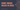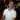# How to Count Unique Values

September 27, 2017

If you ever needed a function in Excel called `CountUnique()`, you might be disappointed to find that no such function exists in Excel. However, there’s a simple trick that we can employ that will do the same thing: `CountIf()`. Let’s take a look.

## How to Count Unique Values using CountIf and Sum

Here is the formula we’ll be working with:

``=SUM(1/COUNTIF(range,range))``

Where `range` is the same range, applied twice to the `CountIf` function.

Also, this is an array formula, and should be entered with `Ctrl+Shift+Enter` (`Cmd+Shift+Enter` on Mac). To learn more about array formulas, click here.

Let’s say that we have this simple table called `Logs`:

How can we count unique values using this formula? Here’s what the formula looks like:

``{=SUM(1/COUNTIF(Logs[Log Type],Logs[Log Type]))}``

With a result of `2`.

Notice that for the `COUNTIF()` function I repeat the same range. Also, if you’re wondering about the syntax, please see my post about Excel Tables and Structured References.## How this Works

Let’s break this down piece-by-piece to understand what’s going on here.

Since this is an array function, the `COUNTIF(range,range)` portion looks something like this:

First, evaluate the table reference to ranges:

``COUNTIF(A2:A5,A2:A5)``

Next, evaluate the ranges to their values in an array format:

``COUNTIF({"Info","Diagnostic","Info","Info"},{"Info","Diagnostic","Info","Info"})``

Note that the first parameter is the range being checked and the second parameter is the set of values that Excel will check for equality.

The first round will look something like this:

``COUNTIF({"Info","Diagnostic","Info","Info"},{"Info","Diagnostic","Info","Info"})``

The first `Info` on the left was found 3 times on the right.

``````COUNTIF({"Info","Diagnostic","Info","Info"},{"Info","Diagnostic","Info","Info"})
----                                ----                ----   ----

Result:
COUNTIF({3,"Diagnostic","Info","Info"},{"Info","Diagnostic","Info","Info"})
-``````

Then, `Diagnotic` was found `1` time on the right:

``````COUNTIF({3,"Diagnostic","Info","Info"},{"Info","Diagnostic","Info","Info"})
----------                          ----------

Result:
COUNTIF({3,1,"Info","Info"},{"Info","Diagnostic","Info","Info"})
-``````

Then, `Info` was found `3` times again on the right.

``COUNTIF({3,1,3,"Info"},{"Info","Diagnostic","Info","Info"})``

And again on the last `Info`:

``COUNTIF({3,1,3,3},{"Info","Diagnostic","Info","Info"})``

The resulting amount of `Counts` is the following array:

``{3,1,3,3}``

You can think of this as a table:

Log Type Found X Times
Info Found 3 times
Diagnostic Found 1 time
Info Found 3 times
Info Found 3 times

Let’s bring this back to our main function:

``{=SUM(1/{3,1,3,3})}``

The `SUM` function will evaluate to adding all of the numbers in the array together, but it will also make them a fraction:

``(1/3)+(1/1)+(1/3)+(1/3)``

Which equals `2`. Now I hope it makes sense why we needed the `=SUM(1/...)` portion.

Wow, you read the whole article! You know, people who make it this far are true learners. And clearly, you value learning. Would you like to learn more about Excel? Please consider supporting me by buying me a coffee (it takes a lot of coffee to write these articles!).Written by Joseph who loves teaching about Excel.